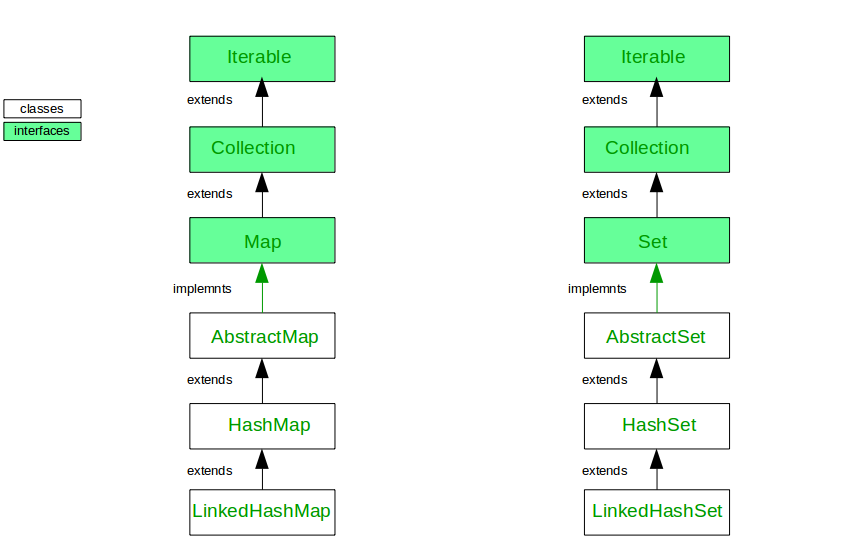• Difficulty Level : Easy
• Last Updated : 16 Sep, 2021

The LinkedHashMap is just like HashMap with an additional feature of maintaining an order of elements inserted into it. HashMap provided the advantage of quick insertion, search, and deletion but it never maintained the track and order of insertion which the LinkedHashMap provides where the elements can be accessed in their insertion order.

Example:

## Java

 `// Java program to demonstrate` `// working of LinkedHashMap` `import` `java.util.*;`   `class` `LinkedHashMapExample {`   `    ``public` `static` `void` `main(String args[])` `    ``{`   `        ``// create an instance of LinkedHashMap` `        ``LinkedHashMap lhm;` `        ``lhm = ``new` `LinkedHashMap();`   `        ``// insert element in LinkedHashMap` `        ``lhm.put(``100``, ``"Amit"``);`   `        ``// insert first null key` `        ``lhm.put(``null``, ``"Ajay"``);` `        ``lhm.put(``101``, ``"Vijay"``);` `        ``lhm.put(``102``, ``"Rahul"``);`   `        ``// insert second null key` `        ``// which replace first null key value` `        ``lhm.put(``null``, ``"Anuj"``);`   `        ``// insert duplicate` `        ``// which replace first 102 key value` `        ``lhm.put(``102``, ``"Saurav"``);`   `        ``// iterate and print the key/value pairs` `        ``lhm.entrySet().stream().forEach((m) -> {` `            ``System.out.println(m.getKey() + ``" "` `                               ``+ m.getValue());` `        ``});` `    ``}` `}`

Output

```100 Amit
null Anuj
101 Vijay
102 Saurav```

The LinkedHashSet is an ordered version of HashSet that maintains a doubly-linked List across all elements. When the iteration order is needed to be maintained this class is used. When iterating through a HashSet the order is unpredictable, while a LinkedHashSet lets us iterate through the elements in the order in which they were inserted. When cycling through LinkedHashSet using an iterator, the elements will be returned in the order in which they were inserted.

Example:

## Java

 `// Java program to demonstrate` `// working of LinkedHashSet` `import` `java.util.*;`   `class` `LinkedHashSetExample {`   `    ``public` `static` `void` `main(String args[])` `    ``{` `        ``// create an instance of LinkedHashSet` `        ``LinkedHashSet lhs` `            ``= ``new` `LinkedHashSet();`   `        ``// insert element in LinkedHashMap` `        ``lhs.add(``"Amit"``);`   `        ``// insert first null key` `        ``lhs.add(``null``);` `        ``lhs.add(``"Vijay"``);` `        ``lhs.add(``"Rahul"``);`   `        ``// insert second null key` `        ``// which replace first null key value` `        ``lhs.add(``null``);` `        ``// insert duplicate` `        ``lhs.add(``"Vijay"``);`   `        ``// create an iterator` `        ``// iterate and print the elements` `        ``Iterator itr = lhs.iterator();` `        ``while` `(itr.hasNext()) {` `            ``System.out.println(itr.next());` `        ``}` `    ``}` `}`

Output

```Amit
null
Vijay
Rahul```